# Materials and Components – Section 3(Page 5)

Materials and Components – Section 3

41. In carbon resistor colour code, if the third band is of silver, it indicates
 A. that three zeros are to be added to indicate the resistance value B. multiplier is 0.01 C. multiplier is 0.1 D. tolerance is ± 5%

42.  In a metal, the drift velocity of electrons in the presence of a electric field E is
 A. proportional to E B. proportional to E C. inversely proportional to E D. proportional to E2

Explanation:

Drift velocity is proportional to field strength.

43.  At a temperature of about 1 K, aluminium is a
 A. conductor B. insulator C. photoconductor D. superconductor

44.  An electron is moving in a circular orbit of radius R at angular velocity ω0 in a field free space. If a magnetic field B is created, the velocity of electron ω will be given by the equation
 A.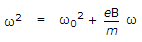B.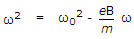C.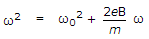D.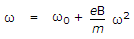45.  If v is the velocity of electron, tc is the average time between collisions, the mean free path λ = vtc.
 A. True B. False

46.  Assertion (A): An electron having charge – e and mass m and lying in an electric field E is subjected to an acceleration – (e/m) E.

Reason (R): If there are n electrons/m3 moving with velocity vx and each electron has a charge – e, the current density J = – n evx.

 A. Both A and R are true and R is correct explanation of A B. Both A and R are true but R is not correct explanation of A C. A is true but R is false D. A is false but R is true

47.  When a pentavalent impurity is added, the semiconductor becomes
 A. p type B. n type C. intrinsic D. neutral

48.  In body centred cubic structure, comers and centre of the cube are occupied by identical atoms.
 A. True B. False

49.  For a multiplate capacitor having n plates, A as area of each plate, the capacitance is proportional to
 A. n A B. (n – 1) A C. (n + 1) A D.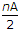Explanation:

If the number of plates is n, the number of capacitors is (n – 1).

50.  The orientation polarization is

1. directly proportional to temperature
2. inversely proportional to temperature
3. inversely proportional to square of permanent dipole moment
4. directly proportional to square of permanent dipole moment.

Which of the above are correct?

 A. 1 and 3 only B. 2 and 3 only C. 1 and 4 only D. 2 and 4 only